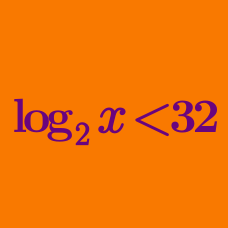Algebra

# Logarithmic Inequalities - Multiple Terms

If the range of $x$ that satisfies ${5}^{\log_{9}x} \cdot {x}^{\log_{9}{5}}-3({5}^{\log_{9}x}+{x}^{\log_{9}{5}})+5 < 0$ is $\alpha < x < \beta,$ what is the value of $\alpha+\beta?$

What is the range of $x$ that satisfies the logarithmic inequality $(\log_{4}x)^2-5\log_{4}x+6 \leq 0 ?$

If the range of $x$ that satisfies the logarithmic inequality $(\log_{5}x)^2-\log_{5}125x^{2} \leq 0$ is $\alpha \leq x \leq \beta,$ what is the value of $\alpha\beta?$

If the logarithmic inequality $(\log_{4}x)^2 \geq \log_{4}ax^2$ is true for all positive numbers $x,$ what is the range of $a ?$

What is the number of integers $x$ that satisfy $\log_{\frac{1}{2}} \frac{4}{x} \cdot \log_{\frac{1}{2}} \frac{x}{8} \geq -2 ?$

×